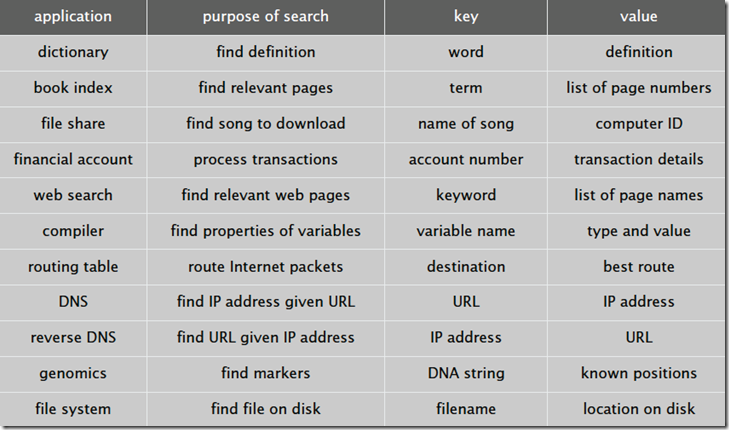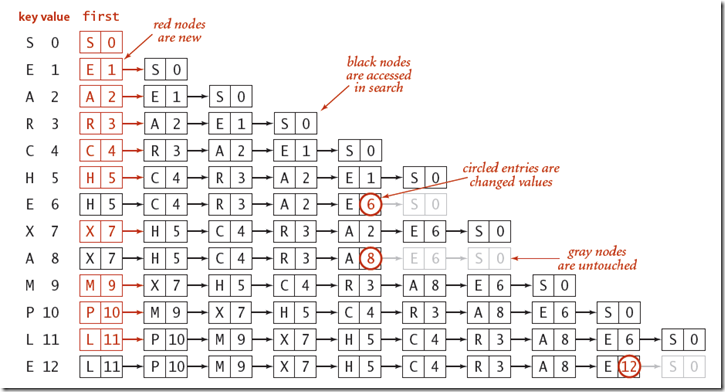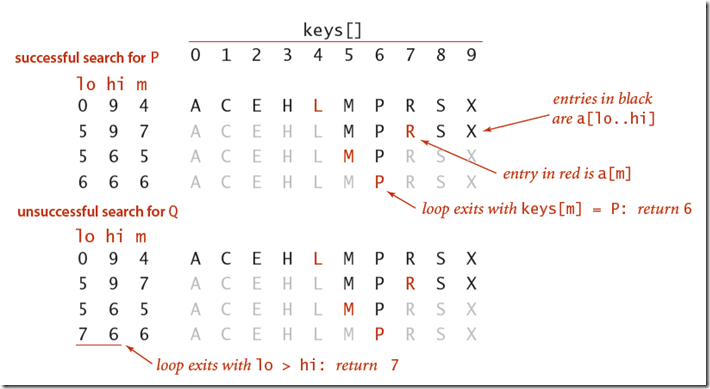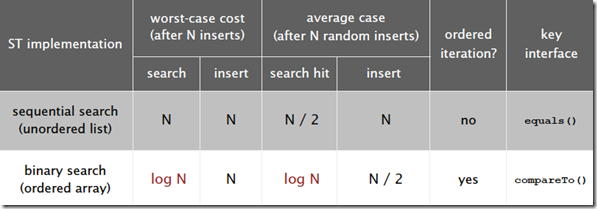# 一符号表public class ST

ST() 创建一个查找表对象
void Put(Key key, Value val) 往集合中插入一条键值对记录，如果value为空，不添加
Value Get(Key key) 根据key查找value，如果没找到返回null
void Delete(Key key) 删除键为key的记录
boolean Contains(Key key) 判断集合中是否存在键为key的记录
boolean IsEmpty() 判断查找表是否为空
int Size() 返回集合中键值对的个数
Iterable Keys() 返回集合中所有的键

# 二实现

## 1 使用无序链表实现查找表``````public class SequentSearchSymbolTable<TKey, TValue> : SymbolTables<TKey, TValue> where TKey : IComparable<TKey>, IEquatable<TKey>
{
private int length = 0;
Node first;
private class Node
{
public TKey key { get; set; }
public TValue value { get; set; }
public Node next { get; set; }

public Node(TKey key, TValue value, Node next)
{
this.key = key;
this.value = value;
this.next = next;
}
}

public override TValue Get(TKey key)
{
TValue result = default(TValue);
Node temp = first;
while (temp != null)
{
if (temp.key.Equals(key))
{
result = temp.value;
break;
}
temp = temp.next;
}

return result;
}

public override void Put(TKey key, TValue value)
{
Node temp = first;
while (temp != null)
{
if (temp.key.Equals(key))
{
temp.value = value;
return;
}
temp = temp.next;
}
first = new Node(key, value, first);
length++;
}

....
}``````

## 2 使用二分查找实现查找表

``````class BinarySearchSymbolTable<TKey, TValue> : SymbolTables<TKey, TValue> where TKey : IComparable<TKey>, IEquatable<TKey>
{
private TKey[] keys;
private TValue[] values;
private int length;
private static readonly int INIT_CAPACITY = 2;
public BinarySearchSymbolTable(int capacity)
{
keys = new TKey[capacity];
values = new TValue[capacity];
length = capacity;
}
public BinarySearchSymbolTable() : this(INIT_CAPACITY)
{
}
/// <summary>
/// 根据key查找value。
/// 首先查找key在keys中所处的位置，如果在length范围内，且存在该位置的值等于key，则返回值
/// 否则，不存在
/// </summary>
/// <param name="key"></param>
/// <returns></returns>
public override TValue Get(TKey key)
{
int i = Rank(key);
if (i < length && keys[i].Equals(key))
return values[i];
else
return default(TValue);
}

/// <summary>
/// 向符号表中插入key，value键值对。
/// 如果存在相等的key，则直接更新value，否则将该key，value插入到合适的位置
///  1.首先将该位置往后的元素都往后移以为
///  2.然后再讲该元素放到为i的位置上
/// </summary>
/// <param name="key"></param>
/// <param name="value"></param>
public override void Put(TKey key, TValue value)
{
int i = Rank(key);
if (i < length && keys[i].Equals(key))
{
values[i] = value;
return;
}
//如果长度相等，则扩容
if (length == keys.Length) Resize(2 * keys.Length);

for (int j = length; j > i; j--)
{
keys[j] = keys[j - 1];
values[j] = values[j - 1];
}

keys[i] = key;
values[i] = value;
length++;
}

/// <summary>
/// 返回key在数组中的位置
/// </summary>
/// <param name="key"></param>
/// <returns></returns>
private int Rank(TKey key)
{
int lo = 0;
int hi = length - 1;
while (lo <= hi)
{
int mid = lo + (hi - lo) / 2;
if (key.CompareTo(keys[mid]) > 0) lo = mid + 1;
else if (key.CompareTo(keys[mid]) < 0) hi = mid - 1;
else return mid;
}
return lo;
}
。。。
}``````

``````private int Rank(TKey key, int lo, int hi)
{
if (lo >= hi) return lo;

int mid = lo + (hi - lo) / 2;
if (key.CompareTo(keys[mid]) > 0)
return Rank(key, mid + 1, hi);
else if (key.CompareTo(keys[mid]) < 0)
return Rank(key, lo, hi - 1);
else
return mid;
}``````# 三 总结Do you have any suggestions for improvement?